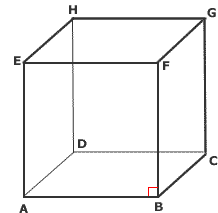# 平行

Lines lying in the same plane but never meeting in either direction.

coplanar straight lines that do not intersect at any point

Two noncoplanar lines are called skew lines.空間中直線關係的基本模型可參照立方體的稜，如右圖，以直線 $$AB$$ 為例：
• 與 $$AB$$ 平行：$$EF$$、$$HG$$、$$DC$$
• 與 $$AB$$ 垂直：$$AD$$、$$AE$$、$$BC$$、$$BF$$
• 與 $$AB$$ 歪斜：$$EH$$、$$FG$$、$$DH$$、$$CG$$

to construct a line parallel to a given line through a given point

The distance between two points of intersection of the parallel lines and a common perpendicular.

A transversal is a line that passes through two parallel lines at two points.

• 同側角 (consecutive angles)，分為同側內角 (consecutive interior angles)、 同側外角 (consecutive exterior angles)。
• 同位角 (corresponding angles)。
• 錯角 (alternate angles)，分為內錯角 (alternate interior angles)、 外錯角 (alternate exterior angles)。

 [語音講解：parallel.mp3]

[ 回上層 ]

Created: Aug 4, 2022
Last Revised: 11/22